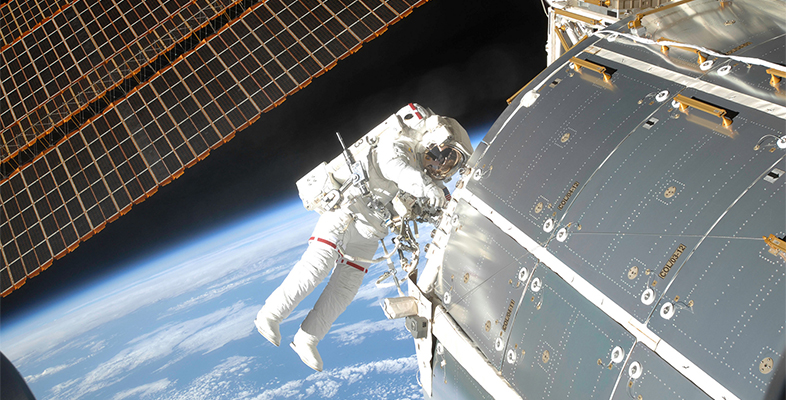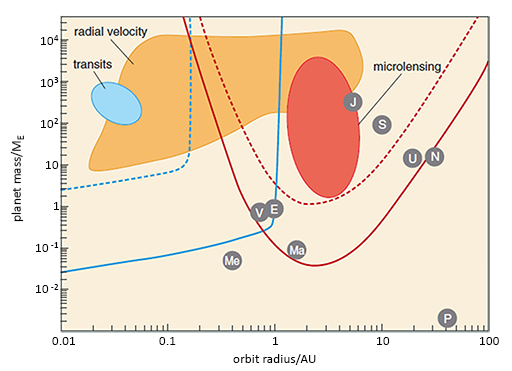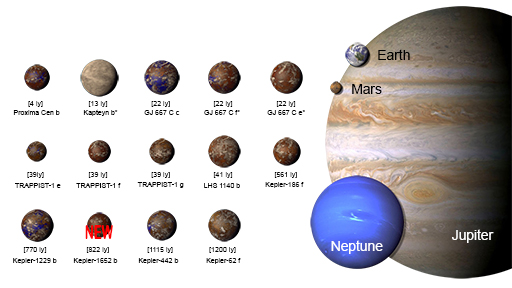Science, Maths & Technology

### Become an OU studentMicrogravity: living on the International Space Station

Start this free course now. Just create an account and sign in. Enrol and complete the course for a free statement of participation or digital badge if available.

# 6 The habitability of planets

There is continuous research into finding exoplanets outside the Solar System with environments similar to Earth’s. But how is this done?

A range of techniques are used. For example, the measurements of planets can be plotted on a graph similar to Figure 6. Here, the orbital radius is measured in terms of astronomical units (AU). This is the distance from the Earth to the Sun (150 million km). The planet’s mass is measured relative to the mass of the Earth (ME) (6 × 1024 kg).

Using Figure 6, now complete Activity 8.Figure 6 A graph used to plot other planets.

## Activity 8 Exploring the habitability of other planets

Timing: Allow approximately 15 minutes

Choose the correct answer to the following questions.

1. Which values are plotted on the horizontal axis?

a.

Orbit

b.

c.

Planet mass

d.

e.

Planet mass/ME

2. Which values are plotted on the vertical axis?

a.

Orbit

b.

c.

Planet mass

d.

e.

Planet mass/ME

3. Where would you expect to find Earth? (Hint: Earth is at a distance of 1 AU from the Sun and has a relative mass of 1 ME.

a.

J

b.

S

c.

E

d.

V

e.

N

4. The planets labelled V, E, J, S and N are in our Solar System. Which one has the largest relative mass?

a.

J

b.

S

c.

E

d.

V

e.

N

5. Which planet has the smallest orbital radius?

a.

J

b.

Me

c.

E

d.

V

e.

Ma

What makes planets habitable for humans?

You might have heard of the ‘Goldilocks zone’. This is the zone occupied by the Earth in its orbit about the Sun. This zone is neither too close to the Sun – that is, too hot – nor too far away from the Sun – that is, too cold. So, Earth is located where the conditions are just right for life to exist.

In trying to find other planets which are suitable for humans, the Planetary Habitability Laboratory (PHL) aims to map the habitable Universe. It holds the Habitable Exoplanets Catalogue (HEC) which lists and compares potentially habitable exoplanets (Figure 7). Using this information in Figure 7, now complete Activity 9.Figure 7 Potentially habitable exoplanets ranked by distance from Earth in light years (ly). Kapteyn b, GJ 667 C e and GJ 667 C f are planet candidates.

## Activity 9 Potentially habitable exoplanets

Timing: Allow approximately 15 minutes

Answer the following questions, choosing one option for each.

1. Which planet is closest to Earth?

a.

Trappist-1 f

b.

Kepler-62 f

c.

GJ 667 C c

d.

Proxima Cen b

e.

Kepler-1229 b

2. According to Figure 7 only, what is the greatest distance of an exoplanet from Earth?

a.

1200 ly

b.

770 ly

c.

1115 ly

d.

2000 ly

e.

39 ly

3. Which one of the following is a ‘planet candidate’?

a.

Trappist-1 f

b.

Kepler-62 f

c.

GJ 667 C c

d.

Proxima Cen b

e.

Kepler-1229 b